# How Big Should I Be Proportional Reasoning 7

• Slides: 21How Big Should I Be? Proportional Reasoning 7 th, 8 th, and 9 th Grade MathLearning Objectives: Students will be able to: • • Solve problems using ratios and proportions. Apply knowledge of ratio and proportion to solve relationships between similar geometric figures. Apply a wide variety of problem-solving strategies (identify a pattern, use equivalent representations) to solve problems from within and outside mathematics. Use various types of logical reasoning in mathematical contexts and real-world situations.Fortnite Official Cinematic Trailer. (2017). Retrieved from https: //www. youtube. com/watch? v=qp. Ktzf 5 f. Xn 4 Stop at 1: 20 Do the characters look real? Why or why not? Think about your answer. Then, share with a partner.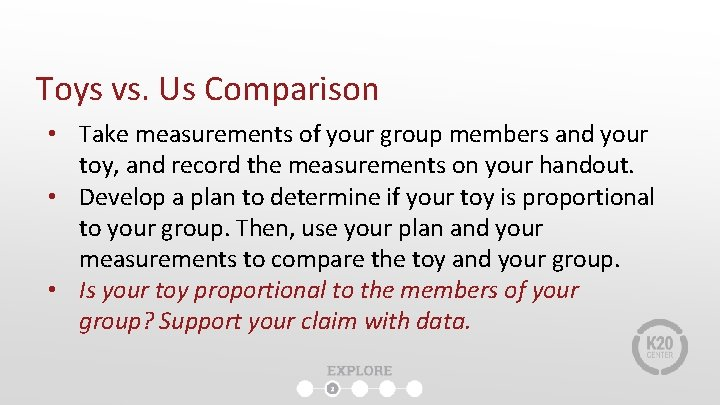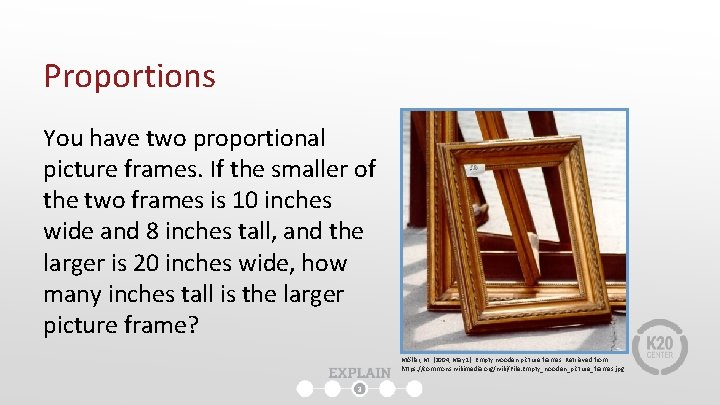Proportions You have two proportional picture frames. If the smaller of the two frames is 10 inches wide and 8 inches tall, and the larger is 20 inches wide, how many inches tall is the larger picture frame? Möller, M. (2004, May 1). Empty wooden picture frames. Retrieved from https: //commons. wikimedia. org/wiki/File: Empty_wooden_picture_frames. jpgProportions You are making a scale model of a blue Studebaker car. The real car is 15 feet long and 9 feet wide. If your scale model were 10 inches long, how wide should it be? Fun Ho! Toy Company. (2017, November 27). Toy car. Retrieved from https: //commons. wikimedia. org/wiki/File: Toy_car_(AM_1996. 165. 108 -1). jpg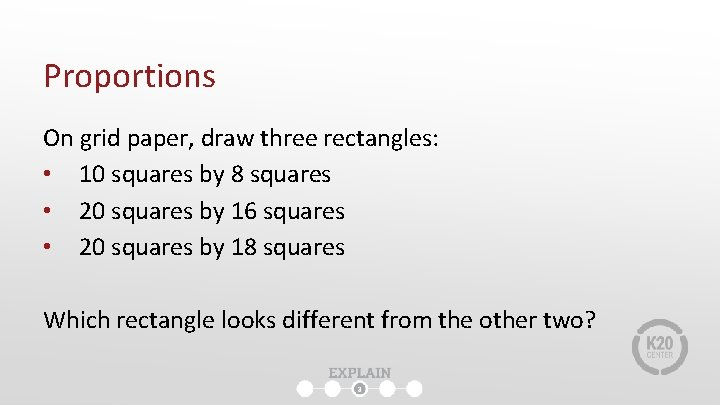Proportions On grid paper, draw three rectangles: • 10 squares by 8 squares • 20 squares by 16 squares • 20 squares by 18 squares Which rectangle looks different from the other two?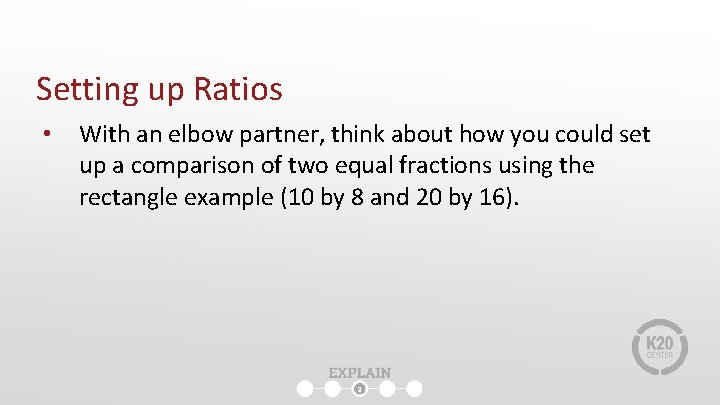Setting up Ratios • With an elbow partner, think about how you could set up a comparison of two equal fractions using the rectangle example (10 by 8 and 20 by 16).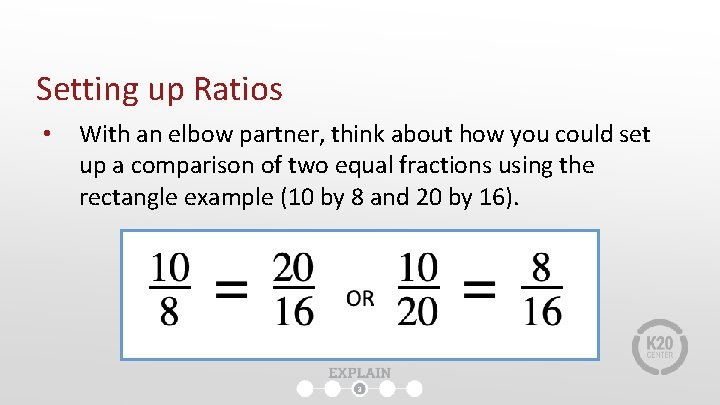Setting up Ratios • With an elbow partner, think about how you could set up a comparison of two equal fractions using the rectangle example (10 by 8 and 20 by 16).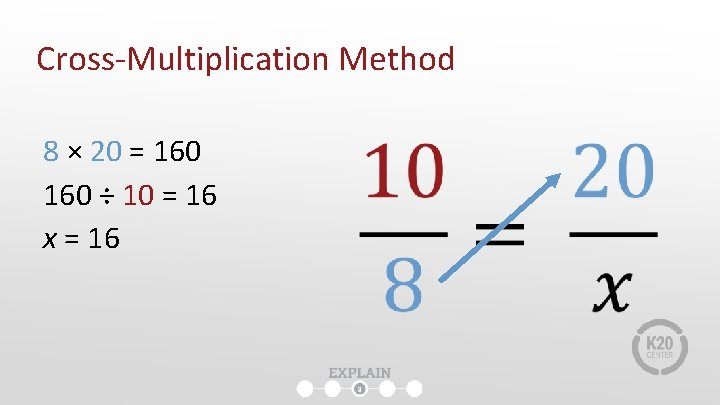Cross-Multiplication Method 8 × 20 = 160 ÷ 10 = 16 x = 16Dilation Rate Method Example 1: 10 × 2 = 20 8 × 2 = 16 x = 16 × 2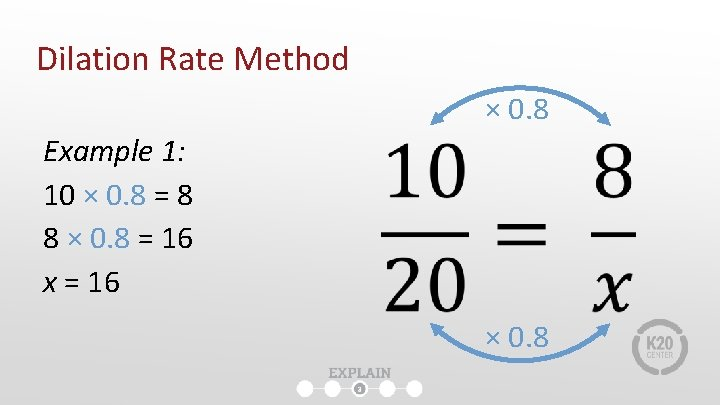Dilation Rate Method × 0. 8 Example 1: 10 × 0. 8 = 8 8 × 0. 8 = 16 x = 16 × 0. 8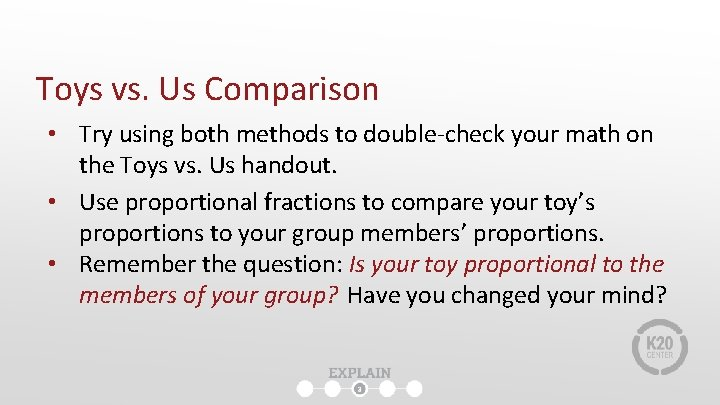Toys vs. Us Comparison • Try using both methods to double-check your math on the Toys vs. Us handout. • Use proportional fractions to compare your toy’s proportions to your group members’ proportions. • Remember the question: Is your toy proportional to the members of your group? Have you changed your mind?Lamilly – Average is Beautiful. (2014). Retrieved from https: //www. youtube. com/watch? v=Of. Qu 8 pq 0 kok#t=58 What are the pros and cons of toy makers and animators using proportions correctly? What about skewing them on purpose?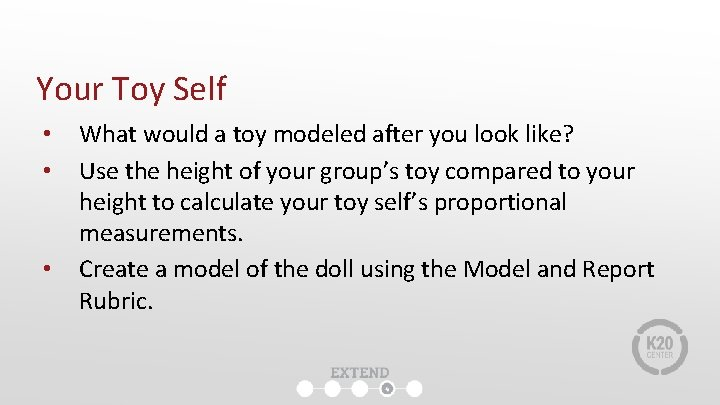Your Toy Self • • • What would a toy modeled after you look like? Use the height of your group’s toy compared to your height to calculate your toy self’s proportional measurements. Create a model of the doll using the Model and Report Rubric.Your Toy Self • Include a written report to answer these questions: ● ● ● What does it mean for two things to be proportional? How do you determine whether two things are proportional? What are three reasons why a toy maker or animator would need to understand the mathematics behind proportions, or understand how to use proportions (or skew them) in their work?